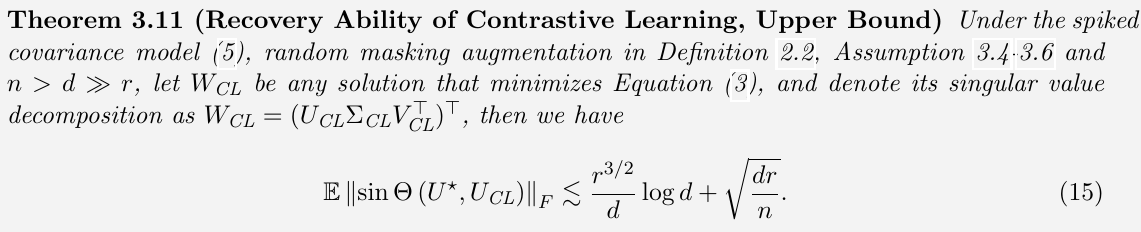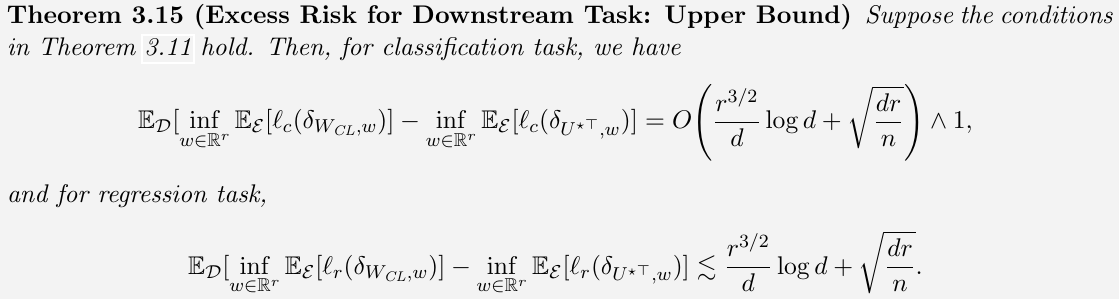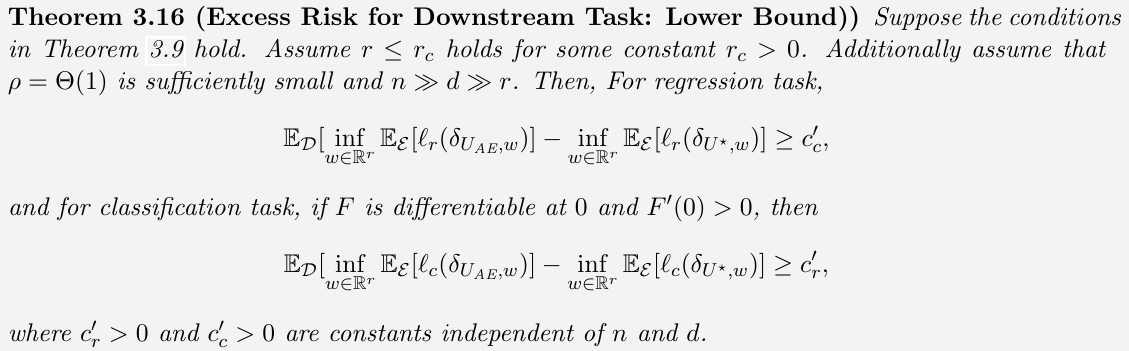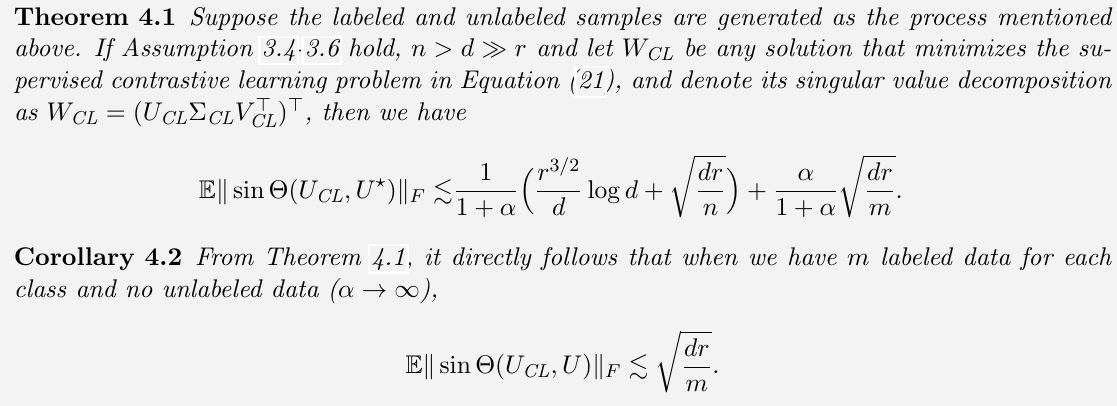# Contrastive Learning: A Simple Framework and A Theoretical Analysis

##### Posted on Oct 06, 2022
Tags: Contrastive Learning

This note is based on

## Simple Framework for Contrastive Learning

• composition of data augmentations plays a critical role in defining effective predictive tasks
• introducing a learnable nonlinear transformation between the representation and the contrastive loss substantially improves the quality of the learned representations
• contrastive learning benefits from larger batch sizes and more training steps compared to supervised learning

Two classes of most mainstream approaches for learning effective visual representations without human supervision:

• generative: learn to generate or otherwise model pixels in the input space
• pixel-level generation is computationally expensive and may not be necessary for representation learning
• discriminative:
• learn representations using objective functions similar to those used for supervised learning, but the inputs and labels are derived from an unlabeled dataset

Four major components:

• stochastic data augmentation
• random cropping followed by resize back to the original size
• random color distortions
• random Gaussian blur
• a neural network base encoder $f(\cdot)$ extracts representation vectors from augmented data examples, use ResNet, $h_i = f(\tilde x_i) = \text{ResNet}(\tilde x_i)$
• a small neural network projection head $g(\cdot)$ that maps representation to the space where contrastive loss is applied, use MLP with one hidden layer $z_i=g(h_i)=W^{(2)}\sigma(W^{(1)}h_i)$. It is beneficial to define the contrastive loss on $z_i$ rather than $h_i$
• a contrastive loss function defined for a contrastive prediction task

## A Theoretical Analysis on Contractive Learning

This is based on Prof. Linjun Zhang’s talk on Ji, W., Deng, Z., Nakada, R., Zou, J., & Zhang, L. (2021). The Power of Contrast for Feature Learning: A Theoretical Analysis (arXiv:2110.02473). arXiv.

• Linear Representation
• Data Generating Process: spiked covariance model
• Self-Supervised Contrastive Learning vs Autoencoders/GANs
• both Autoencoders and GANs are related to PCA
• focus on comparisons between Self-supervised CL vs Autoencoders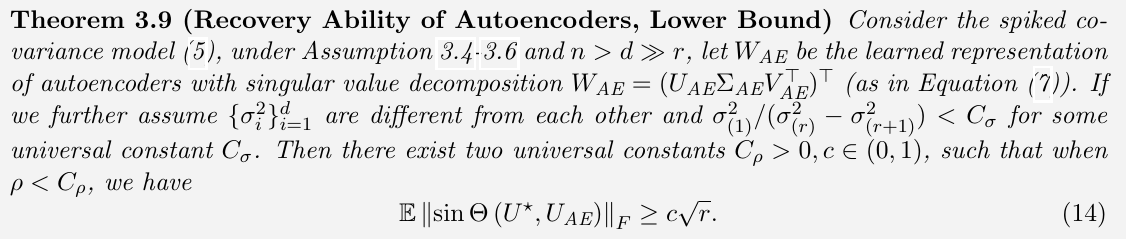it is a constant lower bound, so when $n, d, r$ varies, it is worse than